# Optimizing WBG Devices for High Voltage Applications

//php echo do_shortcode(‘[responsivevoice_button voice=”US English Male” buttontext=”Listen to Post”]’) ?>

Ever since wide bandgap (WBG) materials have been introduced in various manufacturing technologies, it has been possible to achieve high efficiency through power semiconductor devices like MOSFETs, thyristors, and SCRs. Although there have been several developments in silicon carbide (SiC) power diodes and MOSFETS, the optimization of such devices for electrical conductivity remains a major challenge.

In any measurement criterion, the accuracy of the circuit and its output remains an essential aspect. Furthermore, additional complications may arise as device manufacturers do not state critical design parameters on component datasheets.

To optimize controllable manufacturing technology, specific ON-resistance can be used to control most of the power devices in a system. For power MOSFETs, this ON-resistance remains a crucial parameter in optimizing and doping its unit cell design. A major industry standard for maximum electrical conductivity has been the specific ON-resistance versus breakdown (Rsp versus VBD) in material technology.

An experiment was conducted using physics-based static IV and CV measurements to reverse engineer the commercial 4H–SiC JBS power diodes. Once the simulations were performed, it was understood that the commercial 4H–SiC JBS power diodes are rated for punch-through leakage currents. These power diodes are operated at a much lower electric field than the critical electric field strength of the semiconductor.

Apart from this, SiC power diodes have a substantially greater junction capacitance than silicon power diodes. With equal ratings, there is a significant opportunity to enhance the ON-state conductivity of these power devices. The semiconductor industry has significantly tried reducing the defect density in 4H-SiC, but the results have not been too promising. Future research work on this topic must be conducted to enhance the long-term reliability of WBG power devices.

### Constructing diode parameters

Researchers conducted an experiment to test commercial and discrete 4H-SiC JBS power diodes which have ratings from 600 to 1700 V and 1 to 25 A. The devices tested were packaged as per the TO-220 and TO-247 industry standards. To limit the spread of the space charge region beyond punch-through, a buffer layer with higher doping was developed as shown in Figure 1(a) for half-unit cells of vertical JBS diodes. The parameters labeled as P and S indicate the PIN and Schottky diode regions, respectively.Figure 1: Cross-sectional area of ​​half unit cell of a vertical JBS power diode (a) and doping profile used in high voltage 4H-SiC power diode (b). (Source: IEEE)

As shown in Figure 2, the schematic diagram of the electric field distribution at the breakdown has been illustrated. The electric field strength EP.T is at the interface around the drift where the metal-semiconductor interface is during the breakdown period. Both buffer regions and Ec exist because the n-type buffer layer limits the extension of the space-charge region beyond punch-through.

As per the calculated CV characteristics, doping densities are extracted from the drift region and buffer layer as presented in Figure 3.

The calculated normalized zero-bias diode capacitance Cj0N versus normalized total diode area is depicted in Figure 4. Furthermore, a least square line matches the data points, which implies that 600 V diodes were suitable in the rated current along the diode area.

The standard evaluated ON-state characteristics of the diode are shown in Figure 5 (a) and (b) where the diode series resistance (RT), on-voltage (VR), saturation current (IS), and ideality factor (n) are produced. The evaluation performed was conducted under room temperature from 22°C to 250°C for diodes having an extensive range of current ratings from 1 to 25 A along with three different voltage ratings: 600,1200, and 1700 V.Figure 5: (a) Linear scale ON-state characteristics (a) are shown alongside log-linear scale ON-state characteristics (b). (Source: IEEE)

As per extracted values ​​of drift region doping density NDr and punch-through voltage VP.Tthe key diode design parameters are calculated as follows: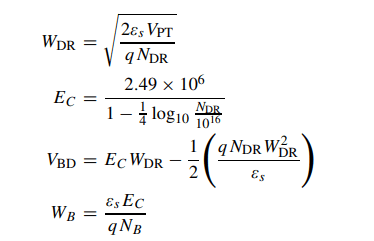From the above equations, Ec is the critical electric field strength for avalanche breakdown, and VBD is the avalanche breakdown voltage. The metal-semiconductor junction built-in potential Vtwo and zero-bias Schottky barrier height φB0I V were computed using the below formulas: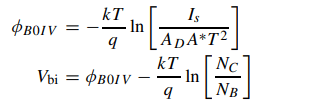The value of A*= 146 A/K2 cm2which is the effective Richardson constant for 4H-SiC, and NC= 3×1015 (T) 3/2 cm-3 shows the effective density of states in the conduction band.

Shown below in Figure 6 (a) and (b) are the extracted values ​​for zero-bias Schottky barrier height (φB0I V) and the diode ideality factor (n) versus the case temperature for 600 V/IA and 1200 V/IA commercial 4H-SiC JBS power diodes are plotted. The graph plotted portrayed the near-ideal diode characteristics and a persistent Schottky contact technology.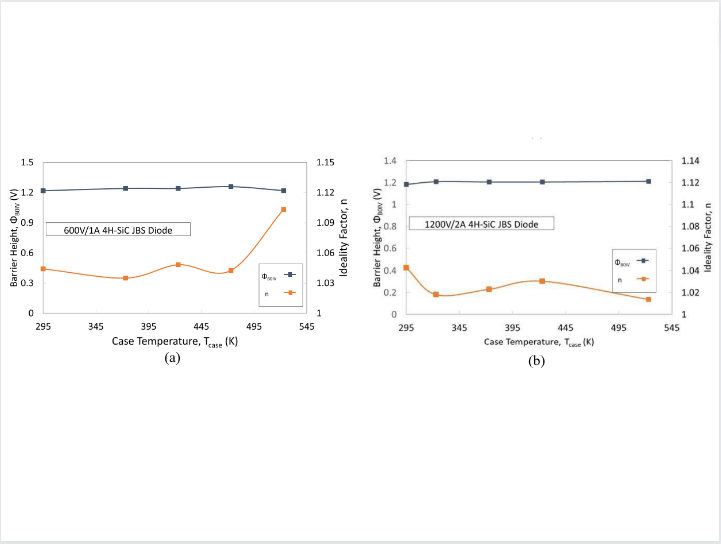Figure 6: Extracted values ​​of zero-bias Schottky barrier height (a) and 1200 V/2 commercial 4H-SiC power diodes (b). (Source: IEEE)

### Calculating drift region resistance

As per the JBS diode structure illustrated in Figure 1, the net drift region resistance RDr can be calculated using the following equation: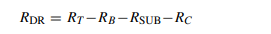As shown in the above formula, there are three resistances involved: RBRSUBand Rc. This includes representing the resistances of the n-type buffer layer, n+ substrate, and cathode metal ohmic contact with the substrate. These resistances are shown below: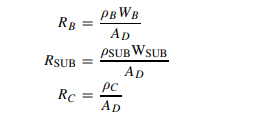As illustrated in the above equation, ρB is the resistivity of the buffer layer that can be easily calculated as the doping density is known. The substrate resistivity ρSUB = 0.012 cm and the substrate thickness WSUB = 377 μm were used in the calculations; for the cathode ohmic contact, a specific contact resistance ρc = 2.5×10−5 cm2 is used. In such cases, even a slight change in the ρc values ​​will affect the results for 600 V devices. A specific drift region resistance RDRS was calculated using the equation below: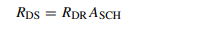The area of ​​the electrically conductive Schottky diode region in Figure 1(a) is ASCH. The calculated results are plotted in Figure 7, where the silicon and 4H-SiC limits are calculated from the above formula. The 4H-SiC JBS diode with VBD= 600 V substrate and cathode ohmic contact resistances contribute largely to the total diode ON-resistance.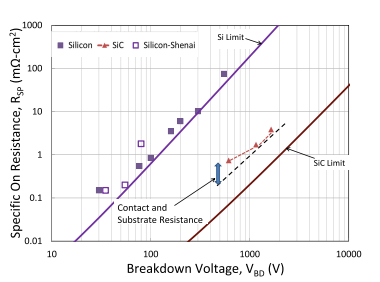Figure 7: Specific ON-resistance is shown versus breakdown voltage for silicon and 4H-SiC carrier devices. (Source: IEEE)

For diodes that have VBD greater than 1,200 V, there is a chance for further development in electrical conductivity, which can be satisfied if the diodes are rated for avalanche breakdown voltage rather than punch-through leakage currents. To achieve this, the buffer layer must be reduced or eliminated entirely by reducing crystal defects in the drift layer.

### Designing reverse leakage currents

The reverse leakage current IL in a Schottky diode comprises of two main components that are as follows:Here, VR is the magnitude of the applied reverse bias voltage and ISCH is the classical thermionic emission current.

The Figure 8 (a) and (b) below illustrate the typical reverse IV characteristics collected from theoretical analysis and experimental readings.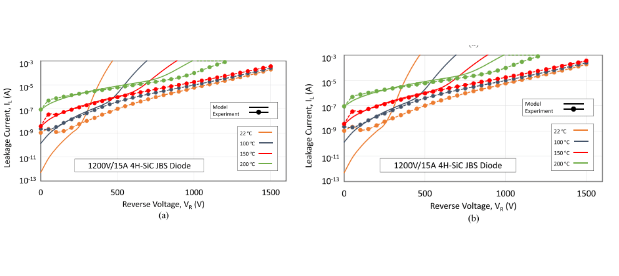Figure 8: Measured plots of modeled reverse leakage currents: (a) 600 V/10 A (b) 1,200 V/15 A. (Source: IEEE)

As demonstrated from the graphs, the errors in the measurement of reverse bias voltage at lower values ​​are caused by measurement equipment compliance. When it comes to higher values, the tunneling currents are much higher when compared to the actual measurements, hence giving an indication of the viable presence of an interfacial dielectric layer near the metal and 4H-SiC.